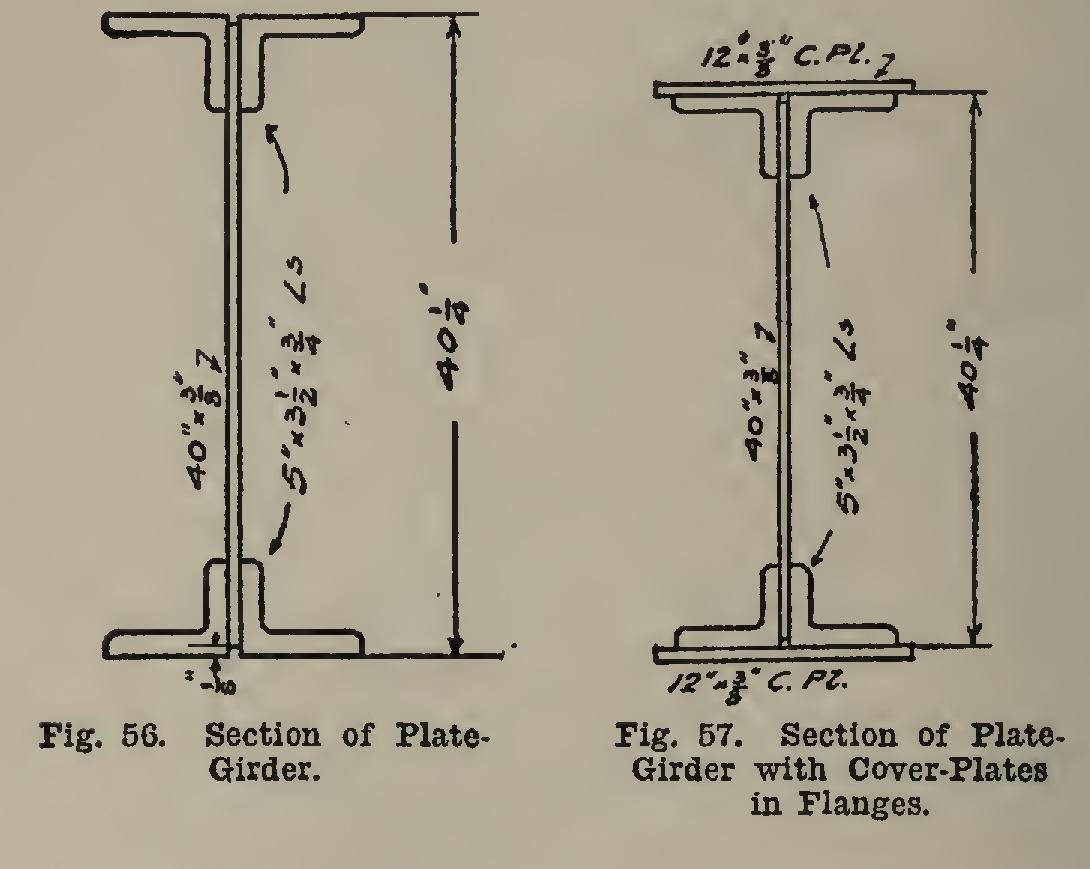# Girders 40

## pounds, weight, angles, girder, inches, web and plate

Page: 1 2 3 4 5 6 7

As an example of the use of the tables, let it be required to design the section of a plate girder which has previously been computed to have a bending moment of 5,330,574 pound inches, the span being 40 feet, and the unit al lowable stress being taken as 15,000 pounds per square inch. If the girder is for railroad or highway use, the depth will usually be stated in the specifications as 1/9 or 1/10 the span or some other ratio. In building operations the depth is usually limited by the head-room re quired in the floor below, or by some other con dition of construction.

Suppose this was a building girder, and the depth was limited to 40 inches. Assume a 5 by angle to be used. The assuming of this angle is a matter of experience. A good guide, however, is to divide the bending moment as given by the allowable unit-stress, and then divide this by the depth chosen, less two inches. The result will then be somewhere near the re quired area a or b of Table XI. In this case, (5,330,57445,000)ũ(40-2)=9.35.

From Table XI it is seen that perhaps either a pair of 5 by by angles or two 5 by by 7/16-in. angles, with a 12 by cover plate, may be used. Two 5 by by 11/16-in. angles give an area very close, but the weight of the girder will increase the above moment, and hence the value a or b. This computation shows that the value can fall in the 5 by angles.

A design will first be made without a cover plate. Then, a=(5,330,574ũ15,000)ũ(40-1.83)=9.31 square inches.

When the weight of the girder is considered, the required area will be greater than this. For the purpose of making an estimate of weight, the angles which give areas next greater than this value are to be used. They are 5 by by and weigh, (C 111), column 4, 19.8 pounds per linear foot for each angle, or 2 x19.8=39.6 pounds per linear foot for each flange. Since there are two flanges, these weigh 2X39.6=79.2 pounds per linear foot of girder. The girder is 40 feet long; and therefore the weight due to the flanges is 79.2>C40=3,168.0 pounds. To this must be added the weight of the web plate. The web plate is designed in a manner similar to that given on page 188. It will be supposed that the greatest reaction is 22,210 pounds, which would be the case if one load of 44,420 pounds was at the center of the span, causing the moment of 5,330,574 pound-inches. The area required in

the web is then: 22,210 =2.22 square inches, the allowable unit-stress being taken as 10,000 pounds per square inch. The required thickness is: 2.22 40 .0.055 inch.

The web will be made inch thick, as explained on page 204. The web is now a plate 40 inches wide and inch thick. From (C 259) the weight given in the 40-inch column and on the line is 51.00 pounds per linear foot. The total weight of the web is then 51.0X40=2,040 pounds. The weight of the flanges and the web ;R.

The weight of the rivet-heads and the stiffener angles amounts to about 1/10 of this, or 5,108ũ 10=511 pounds. The total weight of the girder is now found to be 5,108+511=5,619 pounds; and the maximum moment due to this weight (see page 175) is: 5,619x40x12 8 =337,140 pound-inches.

This must now be added to the amount caused by the loads, thus giving a total of 5,330,574+ 337,140=5,667,714.

The area a is now computed as follows: a=(5,667,714ũ15,000)ũ(40-1.83)=9.899 square inches, which shows, from Table XI, that two 5 by by angles, giving an area of 10.12 inches for a, are the ones for the flange. The section of the girder is shown in Fig. 56.

From an inspection of column 6 of Table XI, it appears as if two 5 by by 7/16-inch angles, with a cover-plate 12 by % inch, may be better; or perhaps two 5 by by %-inch angles, with a cover-plate thicker than % inch, may do. This will now be investigated.

The weight of the girder with a cover-plate will be taken the same. The small difference will not affect the stresses enough to make any difference in the design. The required area is: a=(5,667,714ũ15,000)ũ(40-1.83)=9.899 square inches, This shows that two 5 by 31/2 by 7/16-inch angles and one cover-plate 12 by can be used, the total net area being 9.93 square inches. The web will be the same size. This gives a lighter flange area than that designed without a cover plate.

As a general thing it is better practice to design with a cover-plate, as this not only gives fess flange area, but the cover-plates join the flanges together better, and a more even dis tribution of stress is assured. The cover-plate will extend the full length, and will be on both top and bottom, of course, since both flanges should be made the same.Page: 1 2 3 4 5 6 7